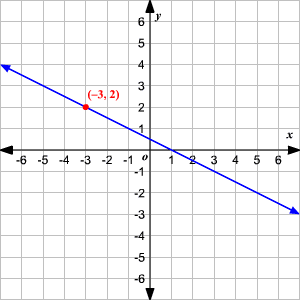# Point-Slope Form

If you know the slope $m$ of a line and the coordinates $\left({x}_{1},{y}_{1}\right)$ of one point on the line, you can write the equation of the line in point-slope form.

$y-{y}_{1}=m\left(x-{x}_{1}\right)$

Example:

Find the equation of a line with slope $-\frac{1}{2}$ passing through the point $\left(-3,2\right)$ .

Here, $m=-\frac{1}{2}$ , ${x}_{1}=-3$ , and ${y}_{1}=2$ , so the equation is:

$y-2=-\frac{1}{2}\left(x-\left(-3\right)\right)$

or

$y-2=-\frac{1}{2}\left(x+3\right)$Slope-intercept form is a special case of point-slope form, where we use the $y$ -intercept coordinate $\left(0,b\right)$ as our point.